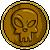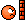# Double Integral

4 replies to this topic

### #1Behnoud

Behnoud

Casio Technician

•• Members
•• 338 posts
• Gender:Male
• Location:Iran

• Calculators:
CP 300+ OS2.2/
FX 3600 pv/
FX 115 s/
FX 602P

Posted 15 June 2007 - 04:20 PM

How to claculate a double integral on a specific surface in CP?
I need to calculate this:
(X^2*Y^2-a^4)/(X^2+Y^2-2*a) on a square surface
which each edge has a length of 2*a and
coordination origin is in center of square, in other hand
the integral limits for both X & Y are from -a to a.
Sorry for my po...or english and thanks in advance.
Btw, It is needed for my exam and is urgent!

### #2Colombia

Colombia

•• Members
•• 94 posts
• Gender:Male
• Location:Venezuela

• Calculators:
algebra FX 2.0 plus

Posted 16 June 2007 - 12:04 AM

I think that you need to study something about Gauss Theorem or Divergence.

if i'm right, you need to calculated the divergence of the function (X^2*Y^2-a^4)/(X^2+Y^2-2*a)
and then calculated the volume of the cubic.

### #3Behnoud

Behnoud

Casio Technician

•• Members
•• 338 posts
• Gender:Male
• Location:Iran

• Calculators:
CP 300+ OS2.2/
FX 3600 pv/
FX 115 s/
FX 602P

Posted 16 June 2007 - 05:18 PM

My math basis is week!### #4PAP

PAP

Casio Overlord

•• Members
•• 681 posts
• Gender:Male
• Location:Somewhere in Europe.
• Interests:Computer Algebra, Numerical Analysis.

• Calculators:
ClassPad 300 (plus an old Casio model, with only a few Kb ram).

Posted 20 June 2007 - 08:53 PM

Two solutions: (1) try to calculate the double integral directly (in CP, you need to calculate the integral of an integral), (2) Convert the double integral to a curvilinear integral using Green's theorem. I suggest to try the first solution; if CP cannot compute the double integral directly. try the second solution.

### #5Behnoud

Behnoud

Casio Technician

•• Members
•• 338 posts
• Gender:Male
• Location:Iran

• Calculators:
CP 300+ OS2.2/
FX 3600 pv/
FX 115 s/
FX 602P

Posted 21 June 2007 - 08:59 AM

It can calculate the integral of an integral,
but if you want it parametrically;
What about calculating on a specific surface for example an triangle?
Btw, I have forgotten Green theorem, Is it time consuming to use it claculate a double integral on CP?

#### 0 user(s) are reading this topic

0 members, 0 guests, 0 anonymous users